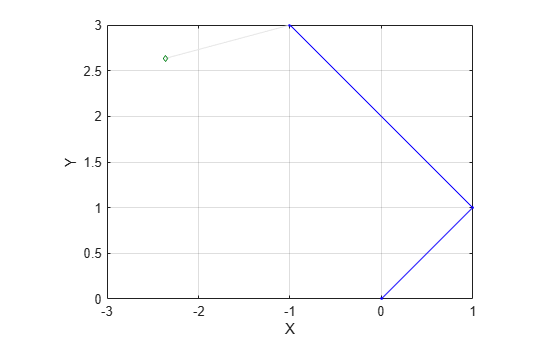Add landmark point node to pose graph

Since R2021a

## Syntax

``addPointLandmark(poseGraph,measurement)``
``addPointLandmark(poseGraph,measurement,infoMat)``
``addPointLandmark(poseGraph,measurement,infoMat,poseNodeID)``
``addPointLandmark(poseGraph,measurement,infoMat,poseNodeID,pointNodeID)``
``[nodePair,edgeID] = addPointLandmark(___)``

## Description

example

````addPointLandmark(poseGraph,measurement)` adds a landmark point node, based on the input position `measurement` that connects to the last pose node in the pose graph. To add pose measurement nodes, see the `addRelativePose` function.```
``` `addPointLandmark(poseGraph,measurement,infoMat)` also specifies the information matrix as part of the edge constraint, which represents the uncertainty of the landmark measurement.```
``` `addPointLandmark(poseGraph,measurement,infoMat,poseNodeID)` adds a new landmark point node and connects it to the pose node specified by `poseNodeID`.```
``` `addPointLandmark(poseGraph,measurement,infoMat,poseNodeID,pointNodeID)` creates an edge by specifying a point measurement between existing nodes, specified by `poseNodeID` and `pointNodeID`. If the node pair already exists, the function appends the new measurement.```
``` `[nodePair,edgeID] = addPointLandmark(___)` returns the newly added edge and edge ID using any combination of inputs from the previous syntaxes. ```

## Examples

collapse all

Create a 2-D pose graph object.

`pg = poseGraph;`

Add relative poses to the pose graph.

```addRelativePose(pg,[1 1 pi/2]); addRelativePose(pg,[2 2 pi/3]);```

Add a landmark point node to the last pose node in the pose graph.

```addPointLandmark(pg,[1 1]); show(pg);```List all poses in the pose graph.

`nodeEstimates(pg)`
```ans = 4×3 0 0 0 1.0000 1.0000 1.5708 -1.0000 3.0000 2.6180 -2.3660 2.6340 NaN ```

## Input Arguments

collapse all

Pose graph, specified as a `poseGraph` or `poseGraph3D` object.

Relative position of the landmark point, specified as one of the following:

For `poseGraph` (2-D), the pose is a two-element vector of form of the form `[x y]`, which defines an xy-position for the landmark.

For `poseGraph3D`, the pose is a three-element vector of the form `[x y z]`, which defines an xyz-position for the landmark.

Information matrix for the landmark, specified as a three-element or six-element vector.

Each vector is the compact form of the upper triangle of the square information matrix. An information matrix represents the uncertainty of the measurement. The matrix is calculated as the inverse of the covariance. If the measurement is an `[x y]` vector, the covariance matrix is a 2-by-2 matrix of pairwise covariance calculations. Typically, the uncertainty is determined by the sensor model.

For `poseGraph` (2-D), each information matrix is a three-element vector. The default is `[1 1 0]`.

For `poseGraph3D`, each information matrix is a six-element vector. The default is `[1 0 0 1 0 1]`.

Pose node to attach from, specified as a positive integer. This integer corresponds to the node ID of a pose node in `poseGraph`. When specified without the `pointNodeID` input, `addPointLandmark` creates a new landmark point node and adds an edge between the new node and the `poseNodeID` node.

Landmark point node to attach to, specified as a positive integer. This integer corresponds to the ID of a landmark node in the pose graph. See the LandmarkNodeIDs property of the pose graph.

## Output Arguments

collapse all

Edge node pair in the pose graph, returned as a two-element vector that lists the IDs of the two nodes that the edge connects. Multiple edges may exist between the same pair of nodes.

ID of added edge, returned as a positive integer.

## Version History

Introduced in R2021a